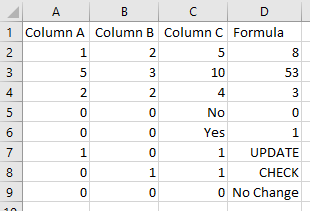###### Technology Solutions for Everyday Folks# Formulas in CSV Files

• August 10, 2020
• 4 minutes

A while back I received a call for assistance in helping to streamline an inherited process. In this particular case, an individual had created a complex (but necessary) process to essentially transform and move data between disparate systems. The employee(s) responsible for creating this process had since left, but the process remained and needed to be manually done often enough that it was painful enough for the person now responsible for the process to ask for assistance. A colleague and I sat in on a virtual meeting during which we watched and listened to all the various steps that were required to see if we could provide any assistance or guidance.

Ultimately we recommended several changes in process, including some we were able to better semi-automate. We needed to maintain a balance of change in production, since this particular process was in mid-cycle (live/production), so the goal was to note items to change more dramatically once the business cycle completes (in another month or so), but implement enough minor changes to help the process be more efficient in the short term.

My role in this process was to address post-processing data from the source system and ship it to a new pickup destination. My colleague's role was to automate a drop of data from the source system. It's important to note that the final step still requires user intervention (loading a file to do the "last mile" work). Along this path of discovery, I asked the relatively simple question:

Can I embed proper Excel/Google Sheets formulas into a CSV (or text) file and have Excel/Google Sheets properly render them on user load?

You can!

### Simple CSV Magic

As it turns out, for the simplest of formulas like basic algebra or SUM, one doesn't even need to encapsulate or escape them in quotes:

``````Column A, Column B, Column C, Formula
1,2,5,=SUM(A2:C2)
5,3,10,=A3*C3+B3
2,2,4,"=COUNTIF(A:C,2)"``````

Rendering that CSV file in Excel or Google sheets will properly calculate and display the formula results, in this case the values `8` and `53` for the `Formula` column of rows two and three. I added an escaped formula for row four (the `COUNTIF`), and it too properly calculates the value `3`.

### Wait, What About More Complex Formulas?

More complex formulas can also be accommodated, but they require some quote-escaping to function properly. This is fairly non-trivial to get working correct, and ultimately the reason I'm writing this post...for future me.

As a basic example of a more complex formula, we'll use an IF statement:

``````Column A, Column B, Column C, Formula
0,0,No,"=IF(and(A5=0,B5=0,C5=""Yes""),1,0)"
0,0,Yes,"=IF(and(A6=0,B6=0,C6=""Yes""),1,0)"``````

In this case, the `Formula` column value for rows two and three will be `No` and `Yes`, respectively. Notice how the text match for "Yes" must be properly escaped with double quotes.

In a more gnarly example, you can do much more (nesting/chaining, operators, etc.):

``````Column A, Column B, Column C, Formula
1,0,1,"=IF(AND(A7=1,B7=0),""UPDATE"",IF(AND(A7=0,B7=1),""CHECK"",""No Change""))"
0,1,1,"=IF(AND(A8=1,B8=0),""UPDATE"",IF(AND(A8=0,B8=1),""CHECK"",""No Change""))"
0,0,0,"=IF(AND(A9=1,B9=0),""UPDATE"",IF(AND(A9=0,B9=1),""CHECK"",""No Change""))"``````

The rendered output for the gnarlier `IF` in the `Formula` column for rows two through four will be `UPDATE`, `CHECK`, and `No Change`, respectively.

In production, because of what we inherited, there were some fairly lengthy and complex formulas...all of which work just as expected when the text values are properly escaped. An example screenshot of all these formulas together:I have a gist of the aforementioned if you're interested in playing around on your own. It's pretty rad!

### Huh. Who Knew?

At the end of the day this is a bit of a niche solution, because there are reasons why one wouldn't normally use a flat text file with formulas (if the user is going to open something, might as well ship a regular file). Additionally, if you're using a CSV/text file to ship data the destination system would need to be able to handle this data, which is far from guaranteed. So it's not necessarily an appropriate mechanism for many situations, but it's super cool that it works and is an easy or bridge mechanism between tech.

As far as next steps on this particular improvement project, one of the key improvements once we're out of the business cycle in question is to actually re-create the logic of the several formulas in the sourcing mechanism (export), which will eliminate the need to fiddle with formulas during the next cycle. But it also requires much more testing and QA work, and thus time. So embedded formulas for the win in the short term!